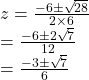## use the discriminant to determine the number of solutions to the quadratic equation −6z2−10z−3=0. What are the real solutions and complex so

Question

use the discriminant to determine the number of solutions to the quadratic equation −6z2−10z−3=0. What are the real solutions and complex solutions?

in progress 0
2 months 2021-07-28T08:33:19+00:00 1 Answers 2 views 0

Step-by-step explanation:

-6z²-10z-3=0

multiply by -1

6z²+10z+3=0

disc .=b²-4ac=10²-4×6×3=100-72=28≥0

also it is not a perfect square.

so roots are real,irrational and different.